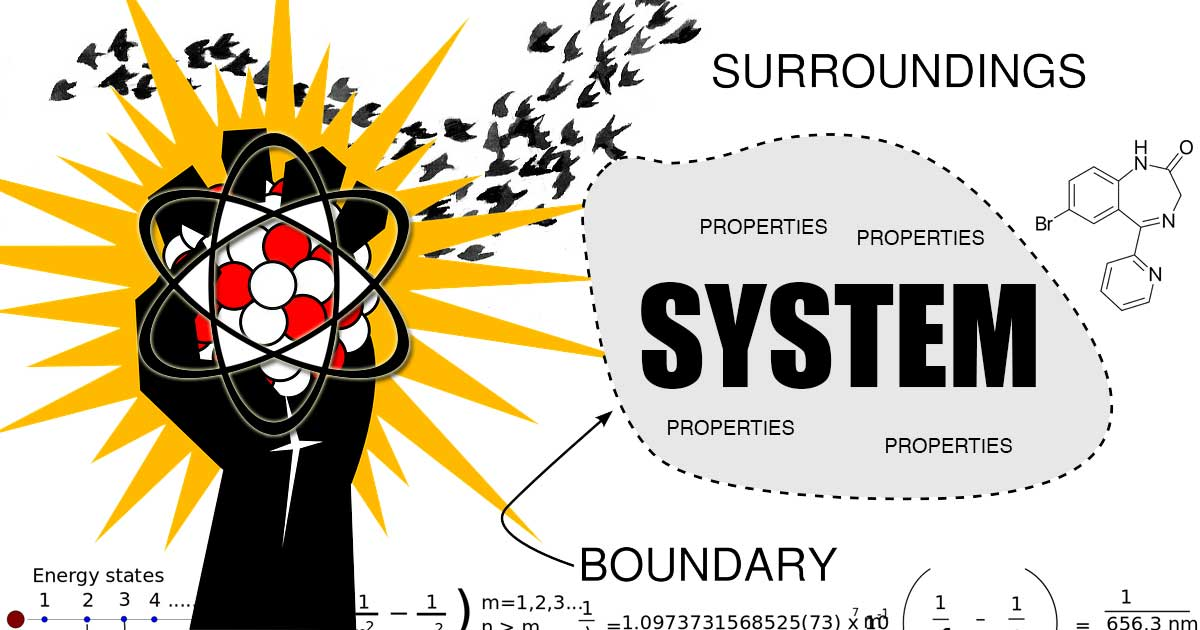# Entropy is the Move From Order to Disorder fact## Entropy, a Measurement of the Move From Order to Disorder in Different Types of Systems

Entropy can be defined more than one way, but in simple terms it can be defined as a the move from order to disorder (the move from potential to equilibrium).

This definition is a little overly simply, and potentially a little misleading in isolation, but it isn’t wrong [at least as far as I can glean from my research]. In fact, that definition is useful in that it hints at other more complete definitions of entropy.

In statistics, entropy is as a measure of the uncertainty of a system. If 100 boxes can be filled with 100 widgets by an algorithm that places widgets into boxes until each box has exactly 1 widget, then entropy is at a minimum when only 1 box is filled with all 100 widgets, and the move toward filling more and more boxes is entropy. Meanwhile, maximum entropy is when all boxes are filled equally (essentially the opposite state of all data points being in one box). If we started with a random pattern, we would lose all ability to detect what the original pattern was once we got to maximum entropy (as filling each box with 1 widget would leave us with no hints of how we go there).

In thermodynamics a simple definition of entropy is the ability of a system to do useful work. Useful work can be done because the energy of the system is not in equilibrium with the the environment outside the system. Thus, entropy can occur in the system until equilibrium between the two systems is achieved. The move from non-equilibrium to equilibrium is entropy (or, the move from one equilibrium state to another state of equilibrium).

For another example, entropy can be thought in terms of probability. In this sense it is the process of things that have a chance of occurring occurring over time until either nothing more can occur or until all things have an equal chance of occurring (where those things that have a greater chance of occurring will occur until an end-point is reached).

Ice has a chance of absorbing energy when the air around it is warmer (the energy of the air enters the ice, because ice has a lower energy; it is colder). Entropy occurs as chance manifests and energy leaves the air (entropy decreases) and enters the ice (entropy increases). Entropy causes the air to cool and the ice to warm and turn to water (the two systems, the air and water, become the same temperature as equilibrium occurs between the energy levels of the two systems, based on the probability of the energy moving between the systems; entropy).

That is the gist of entropy, the fundamental truth behind all systems with energy and the core of the Second Law of Thermodynamics.

TIP: The singularity before the big bang was like a little point of infinite energy and potential. As it expanded, entropy occurred, and it moved from “order” to disorder as probability after probability played out, and energy dissipated to come into equilibrium with the space surrounding it. The result is countless “things” forming out of the energy as that energy moves outward in all probable directions. It will stop only when the universe reaches its maximum state of entropy.

What is entropy? – Jeff Phillips.

Entropy: Embrace the Chaos! Crash Course Chemistry #20.

1. Second Law of Thermodynamics. NASA.gov.
2. Entropy. Wikipedia.org.
3. Entropy. Dictionary.com